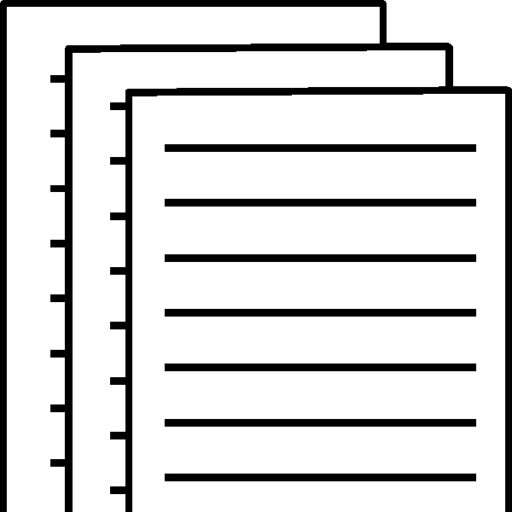## BA215 W7 Written Assignment Week 7 Assignment

Week 7 Assignment

Question 1

Assume you have noted the following prices for paperback books and the number of pages that each book contains.
Develop a least-squares estimated regression line.

Compute the coefficient of determination and explain its meaning.

Compute the correlation coefficient between the price and the number of pages. Test to see if x and y are related. Use α = 0.10.

Develop a least-squares estimated regression line

The regression equation is
y = – 0.49 + 0.0123 x

Coefficient of determination = Rsq = 73.8%

• Compute the coefficient of determination and explain its meaning.

It expresses the proportion of variation in price which is explained by variation in book pages.

Correlation coefficient (r) = =sqrt(Rsq) = sqrt(73.8%) = 0.8591

• Compute the correlation coefficient between the price and the number of pages

The correlation coefficient between price and book pages is 0.8591.

Here the hypothesis for the test is,

• Use the t test to determine whether the slope of the regression model is significant at a= 0.1

H0: B = 0 Vs H1: B not= 0

B is population slope for book pages.

Predictor Coef SE Coef T P
Constant -0.492 1.751 -0.28 0.788
x 0.012295 0.002993 4.11 0.006

S = 0.974399 R-Sq = 73.8% R-Sq(adj) = 69.4%

The test statistic is t = 4.11

P-value = 0.006

P-value < a (0.1)

Reject H0 at 10% level of significance.

The result is significant.

Conclusion: Population slope for book pages is differ than 0.

That is, we can conclude the variable book pages.

Question 2

The following data represent a company’s yearly sales volume and its advertising expenditure over a period of 8 years.Develop a scatter diagram of sales versus advertising and explain what it shows regarding the relationship between sales and advertising.

Use the method of least squares to compute an estimated regression line between sales and advertising.

If the company’s advertising expenditure is \$400,000, what are the predicted sales? Give the answer in dollars.

What does the slope of the estimated regression line indicate?

 variables Intercept Advertising in (\$10,000) confidence interval coefficients std. error t (df=6) p-value 95% lower 95% upper 6.3333 5.9974 1.056 .3316 -8.3419 21.0085 0.3132 0.1594 1.965 .0971 -0.0769 0.7033

To view and download a complete answer, scroll down to the bottom to pay